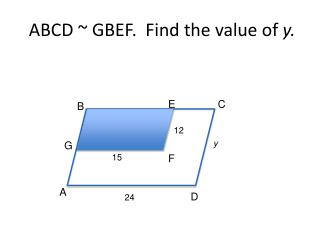DownloadDownload PresentationABCD ~ GBEF. Find the value of y.

ABCD ~ GBEF. Find the value of y.

Télécharger la présentationABCD ~ GBEF. Find the value of y.

- - - - - - - - - - - - - - - - - - - - - - - - - - - E N D - - - - - - - - - - - - - - - - - - - - - - - - - - -
Presentation Transcript

1. ABCD ~ GBEF. Find the value of y. E C B 12 y G 15 F A D 24

2. Quiz Review • Find the scale factor of Figure A to Figure B. • If triangle PQR ~ to triangle STU, then find all possible values of x. B A 4 6 P 7 - x T Q 13 - x U S R

3. Example 2: • Plot the given points on graph paper. Draw quadrilateral ABCD and A’B’. Locate points C’ and D’ so that A’B’C’D’ is similar to ABCD. A(0, 0), B(4, 0), C(2, 4), D(0, 2), A’(-10, -2), B’(-2, -2) C’(-6,6), D’(-10,2) C’(-6,-10), D’(-10,-6)

4. Determine whether each statement is always, sometimes or never • Two congruent triangles are similar • Two squares are similar • A triangle is similar to a quadrilateral • Two isosceles triangles are similar • Two rectangles are similar • Two obtuse triangles are similar • Two equilateral triangles are similar always always never sometimes sometimes sometimes always

5. Graph the given points. Draw polygon ABCD and segment MN. Find the coordinates for vertices L and P such that ABCD~NLPM. A(2,0), B(4,4), C(0,4), D(-2,0), M(4,0), N(12,0) L(16,8) and P(8,8) or L(16,-8) and P(8,-8)

6. State if the polygons are similar and how. 1. 2. Not similar similar

7. Write the similarity statement, and find x, the measures of the indicated sides, and the scale factor. D E 6 12-x C x+7 4 A B

8. Closure • State the similarities and differences of the perimeters and areas of two similar polygons.